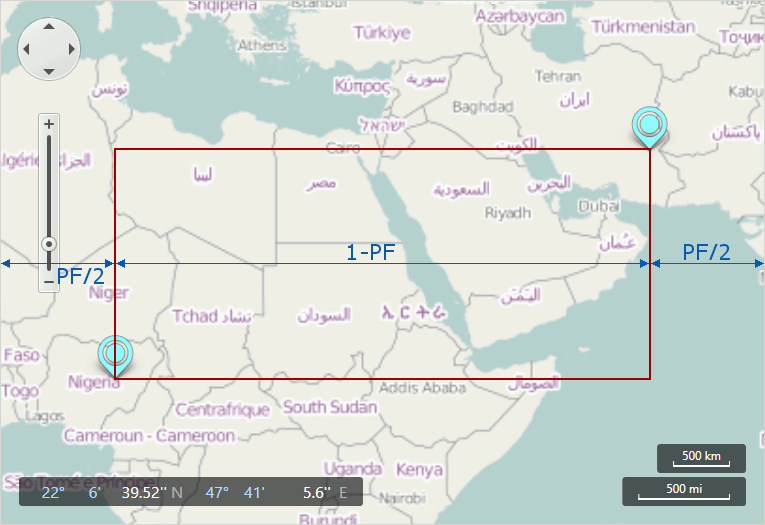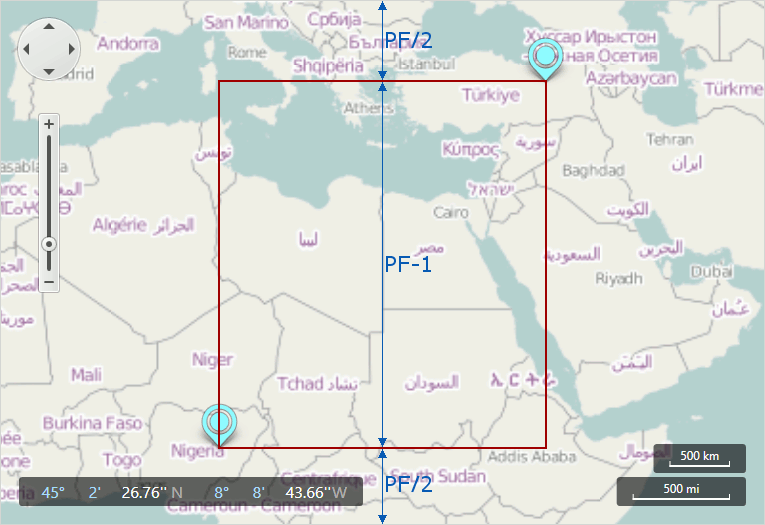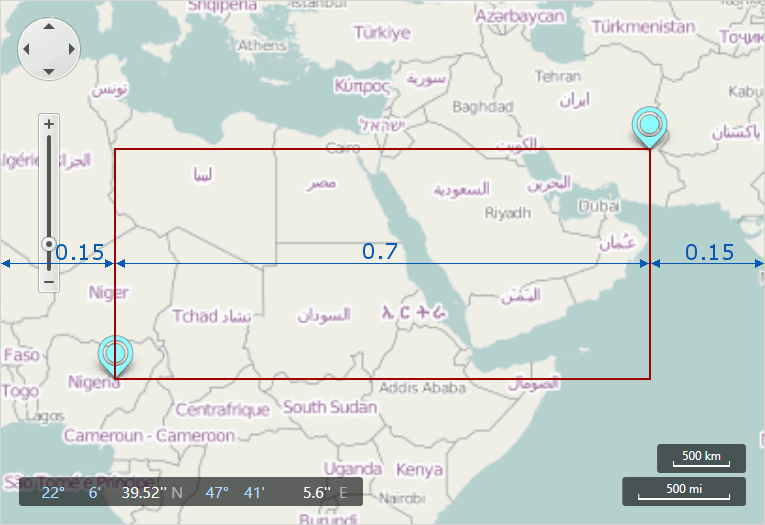20.1
19.2
19.1
18.2
18.1
17.2

# MapControl.ZoomToRegion(CoordPoint, CoordPoint, Double) Method

Zooms to the region described by its top left and bottom right points.

Namespace: DevExpress.Xpf.Map

Assembly: DevExpress.Xpf.Map.v20.1.dll

## Declaration

``````public void ZoomToRegion(
CoordPoint p1,
CoordPoint p2,
)``````
``````Public Sub ZoomToRegion(
p1 As CoordPoint,
p2 As CoordPoint,
)``````

## Parameters

Name Type Description
p1 CoordPoint

A GeoPoint object.

p2 CoordPoint

A GeoPoint object.

## Optional Parameters

Name Type Default Description

0.150000005960464

A Double object, which tunes the border size around the region.

## Remarks

Note that the padding factor is applied to the larger dimension of the region.

The following image shows an instance when the horizontal dimension is larger than the vertical. ("PF" means "Padding Factor" on an image)When vertical dimension larger than the horizontal, the zoom appears as follows.Note that the padding factor is divided by two for each side of the region.

In the instance of the following code

``````GeoPoint p1 = new GeoPoint(10, 10);
GeoPoint p2 = new GeoPoint(30, 60);
mapControl.ZoomToRegion(p1, p2, 0.3);
``````

the result should look like the following.